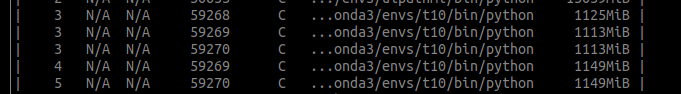# Torchrun using GPU memory on rank 0 for all the processes

For all the processes spanned by torchrun (DDP) a small amount of GPU on rank 0 is used for all the processes.

Test code:

``````import torch
import torch.distributed as dist
import torch.nn as nn
from torch.nn.parallel import DistributedDataParallel as DDP

class ToyModel(nn.Module):
def __init__(self):
super(ToyModel, self).__init__()
self.net = nn.Linear(10, 5)

dist.init_process_group("nccl")
rank = dist.get_rank()
print(f"Start running basic DDP example on rank {rank}.")
# One way to fix it
# import os
# os.environ["CUDA_VISIBLE_DEVICES"] = str(rank)
# torch.cuda.set_device(rank)

device_id = rank % torch.cuda.device_count()
model = ToyModel().to(device_id)
ddp_model = DDP(model, device_ids=[device_id])

if dist.get_rank() == 1:
import pdb; pdb.set_trace()

dist.barrier()
``````

Command used: `OMP_NUM_THREADS=4 torchrun --standalone --nproc_per_node=3 t.py`
Nvidia-smi:There are similar issue posted:

But all of them are because of some where in the code the tensors get allocated to `cuda` which default to rank 0. But it is not the case with the above bare minimal snippet.